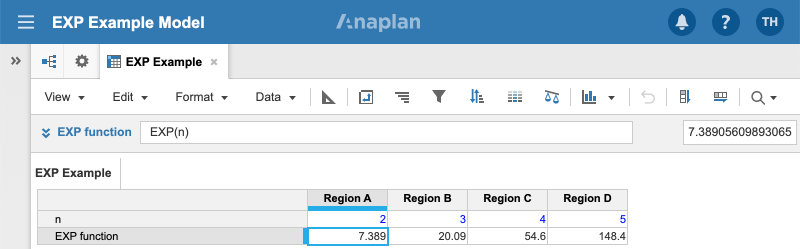1. Calculation functions
2. All Functions
3. Numeric Functions
4. EXPReturns e raised to the nth power, based on the mathematical constant e.

This function is the inverse of the LN function, which returns the logarithm of a number.

## Syntax

`EXP(n) `

where:

• n: The power to raise e to

## Format

Input Format Output Format

n: Number

Number

## Arguments

The function uses the following arguments:

• n: Number: Numeric line item, property, or expression

## Constraints

The function has no constraints.

## Example

The line item EXP function shows the results of the formula:

`EXP(n)  `

The results are:Region list value n Result Region A 2 7.389 Region B 3 20.09 Region C 4 54.6 Region D 5 148.4